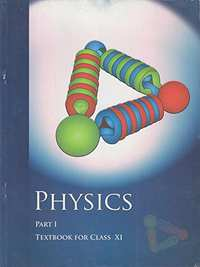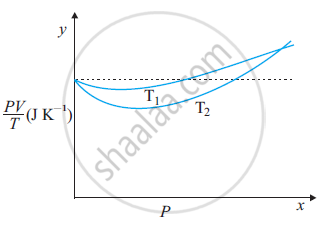# NCERT solutions for Class 11 Physics chapter 13 - Kinetic Theory [Latest edition]

#### Chapters## Chapter 13: Kinetic Theory

Exercises
Exercises [Pages 333 - 335]

### NCERT solutions for Class 11 Physics Chapter 13 Kinetic Theory Exercises [Pages 333 - 335]

Exercises | Q 1 | Page 333

Estimate the fraction of molecular volume to the actual volume occupied by oxygen gas at STP. Take the diameter of an oxygen molecule to be 3Å.

Exercises | Q 2 | Page 333

Molar volume is the volume occupied by 1 mol of any (ideal) gas at standard temperature and pressure (STP: 1 atmospheric pressure, 0 °C). Show that it is 22.4 litres

Exercises | Q 3 | Page 333

The figure shows the plot of PV/versus Pfor 1.00×10–3 kg of oxygen gas at two different temperatures.(a) What does the dotted plot signify?

(b) Which is true: TT2 or T1 < T2?

(c) What is the value of PV/where the curves meet on the y-axis?

(d) If we obtained similar plots for 1.00 ×10–3 kg of hydrogen, would we get the same value of PV/at the point where the curves meet on the y-axis? If not, what mass of hydrogen yields the same value of PV/(for low pressure high temperature region of the plot)? (Molecular mass of H= 2.02 u, of O2 = 32.0 u, = 8.31 J mo1–1 K–1.)

Exercises | Q 4 | Page 334

An oxygen cylinder of volume 30 litres has an initial gauge pressure of 15 atm and a temperature of 27 °C. After some oxygen is withdrawn from the cylinder, the gauge pressure drops to 11 atm and its temperature drops to 17 °C. Estimate the mass of oxygen taken out of the cylinder (= 8.31 J mol–1 K–1, molecular mass of O2 = 32 u)

Exercises | Q 5 | Page 334

An air bubble of volume 1.0 cm3 rises from the bottom of a lake 40 m deep at a temperature of 12 °C. To what volume does it grow when it reaches the surface, which is at a temperature of 35 °C?

Exercises | Q 6 | Page 334

Estimate the total number of air molecules (inclusive of oxygen, nitrogen, water vapour and other constituents) in a room of capacity 25.0 m3 at a temperature of 27 °C and 1 atm pressure

Exercises | Q 7.1 | Page 334

Estimate the average thermal energy of a helium atom at room temperature (27 °C).

Exercises | Q 7.2 | Page 334

Estimate the average thermal energy of a helium atom at the temperature on the surface of the Sun (6000 K).

Exercises | Q 7.3 | Page 334

Estimate the average thermal energy of a helium atom at the temperature of 10 million Kelvin (the typical core temperature in the case of a star).

Exercises | Q 8.1 | Page 334

Three vessels of equal capacity have gases at the same temperature and pressure. The first vessel contains neon (monatomic), the second contains chlorine (diatomic), and the third contains uranium hexafluoride (polyatomic).

Do the vessels contain an equal number of respective molecules?

Exercises | Q 8.2 | Page 334

Three vessels of equal capacity have gases at the same temperature and pressure. The first vessel contains neon (monatomic), the second contains chlorine (diatomic), and the third contains uranium hexafluoride (polyatomic).

Is the root mean square speed of molecules the same in the three cases? If not, in which case is vrms the largest?

Exercises | Q 9 | Page 334

At what temperature is the root mean square speed of an atom in an argon gas cylinder equal to the rms speed of a helium gas atom at – 20 °C? (atomic mass of Ar = 39.9 u, of He = 4.0 u).

Exercises | Q 10 | Page 334

Estimate the mean free path and collision frequency of a nitrogen molecule in a cylinder containing nitrogen at 2.0 atm and temperature 17 °C. Take the radius of a nitrogen molecule to be roughly 1.0 Å. Compare the collision time with the time the molecule moves freely between two successive collisions (Molecular mass of N2 = 28.0 u).

Exercises | Q 11 | Page 335

A metre long narrow bore held horizontally (and closed at one end) contains a 76 cm long mercury thread, which traps a 15 cm column of air. What happens if the tube is held vertically with the open end at the bottom?

Exercises | Q 12 | Page 335

From a certain apparatus, the diffusion rate of hydrogen has an average value of 28.7 cm3 s–1. The diffusion of another gas under the same conditions is measured to have an average rate of 7.2 cm3 s–1. Identify the gas

[Hint: Use Graham’s law of diffusion: R1/R2 = (M2/M1)1/2, where R1, R2 are diffusion rates of gases 1 and 2, and M1 and M2 their respective molecular masses. The law is a simple consequence of kinetic theory.]

Exercises | Q 13 | Page 335

A gas in equilibrium has uniform density and pressure throughout its volume. This is strictly true only if there are no external influences. A gas column under gravity, for example, does not have the uniform density (and pressure). As you might expect, its density decreases with height. The precise dependence is given by the so-called law of atmospheres

n2 = n1 exp [-mg (h– h1)/ kBT]

Where n2, n1 refer to number density at heights h2 and h1 respectively. Use this relation to derive the equation for sedimentation equilibrium of a suspension in a liquid column:

n2 = n1 exp [-mg NA(ρ - P′) (h2 –h1)/ (ρRT)]

Where ρ is the density of the suspended particle, and ρ’ that of surrounding medium. [NA is Avogadro’s number, and R the universal gas constant.] [Hint: Use Archimedes principle to find the apparent weight of the suspended particle.]

Exercises | Q 14 | Page 335

Given below are densities of some solids and liquids. Give rough estimates of the size of their atoms:

 Substance Atomic Mass (u) Density (103 Kg m-3) Carbon (diamond) 12.01 2.22 Gold 197.00 19.32 Nitrogen (liquid) 14.01 1.00 Lithium 6.94 0.53 Fluorine (liquid) 19.00 1.14

[Hint: Assume the atoms to be ‘tightly packed’ in a solid or liquid phase, and use the known value of Avogadro’s number. You should, however, not take the actual numbers you obtain for various atomic sizes too literally. Because of the crudeness of the tight packing approximation, the results only indicate that atomic sizes are in the range of a few Å].

## Chapter 13: Kinetic Theory

Exercises## NCERT solutions for Class 11 Physics chapter 13 - Kinetic Theory

NCERT solutions for Class 11 Physics chapter 13 (Kinetic Theory) include all questions with solution and detail explanation. This will clear students doubts about any question and improve application skills while preparing for board exams. The detailed, step-by-step solutions will help you understand the concepts better and clear your confusions, if any. Shaalaa.com has the CBSE Class 11 Physics solutions in a manner that help students grasp basic concepts better and faster.

Further, we at Shaalaa.com provide such solutions so that students can prepare for written exams. NCERT textbook solutions can be a core help for self-study and acts as a perfect self-help guidance for students.

Concepts covered in Class 11 Physics chapter 13 Kinetic Theory are Specific Heat Capacities - Gases, Mean Free Path, Kinetic Theory of Gases - Concept of Pressure, Equation of State of a Perfect Gas, Work Done in Compressing a Gas, Introduction of Kinetic Theory of an Ideal Gas, Interpretation of Temperature in Kinetic Theory, Gases and Its Characteristics, Assumptions of Kinetic Theory of Gases, RMS Speed of Gas Molecules, Degrees of Freedom, Avogadro's Number, Molecular Nature of Matter, Law of Equipartition of Energy.

Using NCERT Class 11 solutions Kinetic Theory exercise by students are an easy way to prepare for the exams, as they involve solutions arranged chapter-wise also page wise. The questions involved in NCERT Solutions are important questions that can be asked in the final exam. Maximum students of CBSE Class 11 prefer NCERT Textbook Solutions to score more in exam.

Get the free view of chapter 13 Kinetic Theory Class 11 extra questions for Class 11 Physics and can use Shaalaa.com to keep it handy for your exam preparation